2019-11-10 18:13:48 qq_16829085 阅读数 19
• ###### 机器学习之概率与统计推断

本课程讲解机器学习算法所需概率和统计推断知识。概率部分包括概率公理及推论、条件概率、贝叶斯公式、随机变量及其概率函数（CDF／pdf）、常用概率分布及其均值、方差；统计推断部分包括大数定律和中心极限定理、极大似然估计、贝叶斯估计，估计的评价、偏差-方差平衡。课程还会讲解假设检验的基本概念。

20160 人正在学习 去看看 AI100讲师

## 参考资料:

``````'''
@version: 0.0.1
@Author: Huang
@dev: python3 vscode
@Date: 2019-11-10 11:39:30
@LastEditTime: 2019-11-10 17:57:13
@FilePath: \\机器学习实战\\10-K均值聚类算法\\kMeans.py
@Descripttion: 聚类是一种无监督的学习，它将相似的对象归到同一个簇中
'''

import numpy as np
import matplotlib.pyplot as plt

"""
[summary]:加载数据

Arguments:
filename  -- 文件名

Returns:
[List] -- 数据集
"""
dataMat = []
with open(filename) as fr:
curline = line.strip().split()
fltline = list(map(float, curline))
dataMat.append(fltline)
return dataMat

def distEclud(vecA, vecB):
"""
[summary]:计算两个向量的欧氏距离

Arguments:
vecA  -- A坐标
vecB  -- B坐标

Returns:
两点之间的欧氏距离
"""
# not sum(mat) but mat.sum()
return np.sqrt(np.power(vecA - vecB, 2).sum())

def randCent(dataSet, k):
"""
[summary]:为数据集构建k个随机质心的集合

Arguments:
dataSet {[mat]} -- 数据集
k {[int} -- 聚类数

Returns:
[mat] -- k个中心点组成的矩阵
"""
n = np.shape(dataSet)  # 获取列数
centroids = np.mat(np.zeros((k, n)))
# 遍历所有列
for j in range(n):
minj = min(dataSet[:, j])
rangej = float(max(dataSet[:, j]) - minj)
# 最小值+区间×随机系数,确保生成的中心点在数据集边界之内
centroids[:, j] = minj + rangej * np.random.rand(k, 1)
return centroids

def my_KMeans(dataSet, k, distMeas=distEclud, createCent=randCent):
"""
[summary]:
创建k个点作为起始质心（经常是随机选择）
当任意一个点的簇分配结果发生改变时
对数据集中的每个数据点
对每个质心
计算质心与数据点之间的距离
将数据点分配到距其最近的簇
对每一个簇，计算簇中所有点的均值并将均值作为质心

Arguments:
dataSet {[mat]} -- 数据集
k {[int]} -- 聚类数

Keyword Arguments:
distMeas  -- 距离算法 (default: {distEclud})
createCent -- 创建初始质心 (default: {randCent})

Returns:
centroids -- (k,n) 类质心
clusterAssment -- (m,2) 点分配
"""
m = np.shape(dataSet)  # 行数
# 簇分配结果：第一列记录索引值;第二列存储误差,当前点到簇质心的距离
clusterAssment = np.mat(np.zeros((m, 2)))
# 随机生成中心点完成初始化
centroids = randCent(dataSet, k)  # (k,n)
clusterchanged = True
while clusterchanged:
# 假定所有点分配都不发生改变，标记为False
clusterchanged = False
for i in range(m):
cluster_i = clusterAssment[i, 0]  # 取出簇索引值
dismax = np.inf
for j in range(k):
curdis = distEclud(centroids[j, :], dataSet[i, :])
if curdis < dismax:
dismax = curdis
# 更新簇分配结果
clusterAssment[i, :] = j, dismax
if cluster_i != clusterAssment[i, 0]:
clusterchanged = True
print(centroids)
for cent in range(k):
ptsInClust = dataSet[np.nonzero(clusterAssment[:, 0].A == cent)]
# 沿矩阵的列方向进行均值计算
centroids[cent, :] = np.mean(ptsInClust, axis=0)
# 返回所有的类质心与点分配结果
return centroids, clusterAssment

def plt_my_KMeans():
# 对数据进行聚类
centroidsOfData, clusterAssmentOfData = my_KMeans(data, 4)
# 数据集的数量
m = np.shape(data)
# 画出数据的散点图
plt.scatter(data[:, 0].A.reshape(m),
data[:, 1].A.reshape(m),
c=clusterAssmentOfData.A[:, 0].reshape(m))
# 用红色的三角形符号画出聚类中心
plt.scatter(centroidsOfData.A[:, 0],
centroidsOfData.A[:, 1],
c='red',
marker='^')
# 显示图片
plt.show()

def biKmeans(dataSet, k, distMeas=distEclud):
"""
[summary]:二分K-均值算法
将所有点看成一个簇
当簇数目小于k时
对于每一个簇
计算总误差
在给定的簇上面进行K-均值聚类(k=2)
计算将该簇一分为二之后的总误差
选择使得误差最小的那个簇进行划分操作

Arguments:
dataSet {[mat]} -- 数据集
k {[int]} -- 聚类数

Keyword Arguments:
distMeas  -- 距离算法 (default: {distEclud})

Returns:
centroids -- (k,n) 类质心
clusterAssment -- (m,2) 点分配
"""
m, n = np.shape(dataSet)
clusterAssment = np.mat(np.zeros((m, 2)))
# 创建一个初始簇
centroid0 = np.mean(dataSet, axis=0).tolist()
cenList = [centroid0]  # 保留质心
# 计算误差
for j in range(m):
clusterAssment[j, 1] = distMeas(np.mat(centroid0), dataSet[j, :])**2
# 对簇进行划分
while len(cenList) < k:
lowestSSE = np.inf
# 尝试划分每一簇
for i in range(len(cenList)):
#找出正在计算的簇
ptscurrCluster = dataSet[np.nonzero(
clusterAssment[:, 0].A == i), :]
# 对给定簇进行K-均值聚类
centroidMat, splitClustAss = my_KMeans(ptscurrCluster, 2, distMeas)
# 计算划分后的SSE(误差平方和)
ssesplit = np.sum(splitClustAss[:, 1])
# 计算剩余数据集的SSE(误差平方和)
ssenotsplit = np.sum(
clusterAssment[np.nonzero(clusterAssment[:, 0].A != i), 1])
# print(ssesplit, ssenotsplit)

if ssesplit + ssenotsplit < lowestSSE:
bestCentToSplit = i
bestnewCent = centroidMat
# numpy中赋值都是将索引赋值，把数据真正赋值要用copy()
bestClustAss = splitClustAss.copy()
lowestSSE = ssenotsplit + ssesplit

# 更新簇的分配结果
bestClustAss[np.nonzero(
bestClustAss[:, 0].A == 1), 0] = len(cenList)
bestClustAss[np.nonzero(
bestClustAss[:, 0].A == 0), 0] = bestCentToSplit
# print('the bestcenttosplit: ', bestCentToSplit)
# print('len bestclustass: ', len(bestClustAss))
# 要划分的簇的簇心坐标更新为其中一个簇心坐标
cenList[bestCentToSplit] = bestnewCent[0, :].A.reshape(n)
# 另一个簇心坐标要通过append添加进簇心坐标集合里
cenList.append(bestnewCent[1, :].A.reshape(n))
# reassign new clusters, and SSE
clusterAssment[np.nonzero(
clusterAssment[:, 0].A == bestCentToSplit), :] = bestClustAss

return np.mat(cenList), clusterAssment

def plt_biKmeans():
centroids, clusterAssment = biKmeans(data, 3)
# 数据集的数量
m = np.shape(data)
# 画出数据的散点图
plt.scatter(data[:, 0].A.reshape(m),
data[:, 1].A.reshape(m),
c=clusterAssment.A[:, 0].reshape(m))
# 用红色的三角形符号画出聚类中心
plt.scatter(centroids.A[:, 0], centroids.A[:, 1], c='red', marker='+')
# 显示图片
plt.show()

def distSLC(vecA, vecB):
# 使用球面余弦定理计算两点的距离
a = np.sin(vecA[0, 1] * np.pi / 180) * np.sin(vecB[0, 1] * np.pi / 180)
b = np.cos(vecA[0, 1] * np.pi / 180) * np.cos(
vecB[0, 1] * np.pi / 180) * np.cos(np.pi *
(vecB[0, 0] - vecA[0, 0]) / 180)
return np.arccos(a + b) * 6371.0  # pi is imported with numpy

def clusterClubs(numClust=5):
datList = []
lineArr = line.split('\t')
datList.append([float(lineArr), float(lineArr)])
datMat = np.mat(datList)
myCentroids, clustAssing = biKmeans(datMat, numClust, distMeas=distSLC)
fig = plt.figure()
rect = [0.1, 0.1, 0.8, 0.8]
scatterMarkers = ['s', 'o', '^', '8', 'p', 'd', 'v', 'h', '>', '<']
axprops = dict(xticks=[], yticks=[])
# 基于图像创建矩阵
ax0.imshow(imgP)
for i in range(numClust):
ptsInCurrCluster = datMat[np.nonzero(clustAssing[:, 0].A == i), :]
markerStyle = scatterMarkers[i % len(scatterMarkers)]
ax1.scatter(ptsInCurrCluster[:, 0].flatten().A,
ptsInCurrCluster[:, 1].flatten().A,
marker=markerStyle,
s=90)
ax1.scatter(myCentroids[:, 0].flatten().A,
myCentroids[:, 1].flatten().A,
marker='+',
s=300)
plt.show()

if __name__ == '__main__':
clusterClubs(5)
# plt_my_KMeans()
# plt_biKmeans()
``````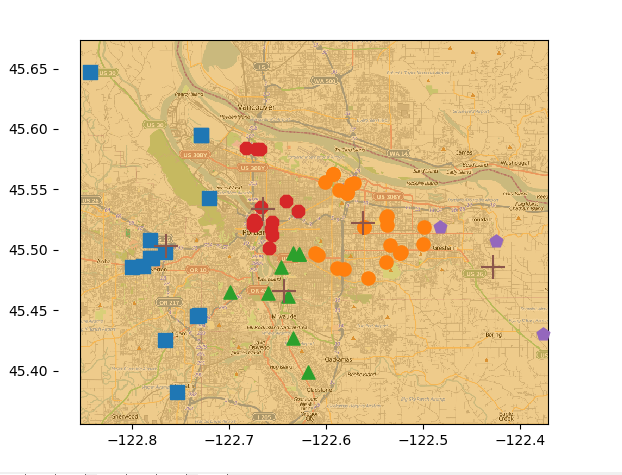k均值 机器学习实战 相关内容

2018-11-01 20:51:16 qq_38662930 阅读数 77
• ###### 机器学习之概率与统计推断

本课程讲解机器学习算法所需概率和统计推断知识。概率部分包括概率公理及推论、条件概率、贝叶斯公式、随机变量及其概率函数（CDF／pdf）、常用概率分布及其均值、方差；统计推断部分包括大数定律和中心极限定理、极大似然估计、贝叶斯估计，估计的评价、偏差-方差平衡。课程还会讲解假设检验的基本概念。

20160 人正在学习 去看看 AI100讲师
```# !/usr/bin/python
# -*- coding:utf-8 -*-

import numpy as np
import matplotlib.colors
import matplotlib.pyplot as plt
import sklearn.datasets as ds
from sklearn.metrics import homogeneity_score, completeness_score, v_measure_score, adjusted_mutual_info_score,\
from sklearn.cluster import KMeans

def expand(a, b):
d = (b - a) * 0.1
return a-d, b+d

if __name__ == "__main__":
N = 400
centers = 4
data, y = ds.make_blobs(N, n_features=2, centers=centers, random_state=2)
data2, y2 = ds.make_blobs(N, n_features=2, centers=centers, cluster_std=(1,2.5,0.5,2), random_state=2)
data3 = np.vstack((data[y == 0][:], data[y == 1][:50], data[y == 2][:20], data[y == 3][:5]))
y3 = np.array( * 100 +  * 50 +  * 20 +  * 5)
m = np.array(((1, 1), (1, 3)))
data_r = data.dot(m)

matplotlib.rcParams['font.sans-serif'] = ['SimHei']
matplotlib.rcParams['axes.unicode_minus'] = False
cm = matplotlib.colors.ListedColormap(list('rgbm'))
data_list = data, data, data_r, data_r, data2, data2, data3, data3
y_list = y, y, y, y, y2, y2, y3, y3
titles = '原始数据', 'KMeans++聚类', '旋转后数据', '旋转后KMeans++聚类',\
'方差不相等数据', '方差不相等KMeans++聚类', '数量不相等数据', '数量不相等KMeans++聚类'

model = KMeans(n_clusters=4, init='k-means++', n_init=5)
plt.figure(figsize=(8, 9), facecolor='w')
for i, (x, y, title) in enumerate(zip(data_list, y_list, titles), start=1):
plt.subplot(4, 2, i)
plt.title(title)
if i % 2 == 1:
y_pred = y
else:
y_pred = model.fit_predict(x)
print(i)
print('Homogeneity：', homogeneity_score(y, y_pred))
print('completeness：', completeness_score(y, y_pred))
print('V measure：', v_measure_score(y, y_pred))
print('Silhouette：', silhouette_score(x, y_pred), '\n')
plt.scatter(x[:, 0], x[:, 1], c=y_pred, s=30, cmap=cm, edgecolors='none')
x1_min, x2_min = np.min(x, axis=0)
x1_max, x2_max = np.max(x, axis=0)
x1_min, x1_max = expand(x1_min, x1_max)
x2_min, x2_max = expand(x2_min, x2_max)
plt.xlim((x1_min, x1_max))
plt.ylim((x2_min, x2_max))
plt.grid(b=True, ls=':')
plt.tight_layout(2, rect=(0, 0, 1, 0.97))
plt.suptitle('数据分布对KMeans聚类的影响', fontsize=18)
plt.show()
```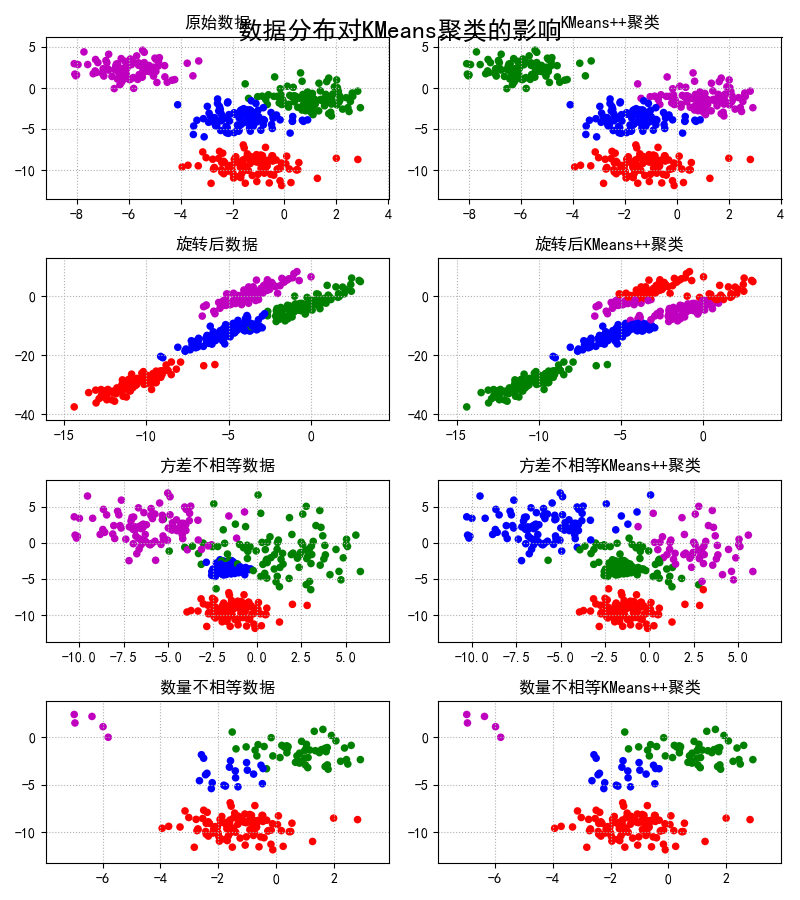k均值 机器学习实战 相关内容

2017-04-15 19:27:51 jiafeier_555 阅读数 1232
• ###### 机器学习之概率与统计推断

本课程讲解机器学习算法所需概率和统计推断知识。概率部分包括概率公理及推论、条件概率、贝叶斯公式、随机变量及其概率函数（CDF／pdf）、常用概率分布及其均值、方差；统计推断部分包括大数定律和中心极限定理、极大似然估计、贝叶斯估计，估计的评价、偏差-方差平衡。课程还会讲解假设检验的基本概念。

20160 人正在学习 去看看 AI100讲师

1 算法思想（1）事先选定K个聚类中心
（2）计算待测样本a到每个聚类中心的距离，然后将样本a分配到距离最近的聚类中
（3）计算每个聚类样本的均值来动态更新原聚类质心
（4）不断迭代（2），（3），直到簇质心不再改变。
2 实现

``````def kMeans(dataSet, k, distMeas=distEclud, createCent=randCent):
m = shape(dataSet) #m=80L
clusterAssment = mat(zeros((m,2)))
#创造一个m个列表，每个列表中2个元素的全零变量
#在matlab中相当于创建一个m行2列的矩阵
#第一列存簇索引值，第二列存当前点到簇质心的距离
centroids = createCent(dataSet, k)  #随机创建k个簇心
clusterChanged = True  #创建标注标量，用来达到条件就终止循环
while clusterChanged:
clusterChanged = False
for i in range(m):#遍历每个样本
minDist = inf  #初始样本到簇质心的距离  nif 表示正无穷；-nif表示负无穷
minIndex = -1
for j in range(k):  #遍历每个簇质心
distJI = distMeas(centroids[j,:],dataSet[i,:])  #计算样本点到簇质心的距离
if distJI < minDist:    #寻找距离最近的簇质心
minDist = distJI
minIndex = j   #将样本分配到距离最小的质心那簇
if clusterAssment[i,0] != minIndex: clusterChanged = True #如果样本分配结果发生变化，更改标志变量
#如果分配后结果不变，就将结果存入clusterAssment，结束while循环
clusterAssment[i,:] = minIndex,minDist**2
print centroids
for cent in range(k):#遍历每个簇质心
#找到每个簇质心对应的样本
ptsInClust = dataSet[nonzero(clusterAssment[:,0].A==cent)]#get all the point in this cluster
centroids[cent,:] = mean(ptsInClust, axis=0) #计算这些样本的均值，作为该簇的新质心
return centroids, clusterAssment``````

3 二分K-均值算法
K-means虽然实现很容易，但却很容易收敛到局部最小，达不到全局最小，所以有人提出二分K-均值。二分K-均值简单来说就是将所有数据集分成两簇，然后再选择误差最小的那个簇再进行二分K-均值划分。3.1 实现

``````def biKmeans(dataSet, k, distMeas=distEclud):
m = shape(dataSet) #60L
clusterAssment = mat(zeros((m,2)))
centroid0 = mean(dataSet, axis=0).tolist() #计算每维的均值  如果axis=1,就是计算每个列表元素的均值
centList =[centroid0] #用每维均值创建初始簇质心,也就是说，将整个数据集当成一簇
for j in range(m):#遍历每个样本
#保存样本到每个簇质心的距离
#所以此处shape(clusterAssment)=（60L,2L）
clusterAssment[j,1] = distMeas(mat(centroid0), dataSet[j,:])**2
while (len(centList) < k):  #如果初始的簇个数小于k
lowestSSE = inf
for i in range(len(centList)):   #遍历初始的那簇
#提取当前簇中的所有样本点
ptsInCurrCluster = dataSet[nonzero(clusterAssment[:,0].A==i),:]
#将这些样本点进行kmeans操作，其中K设为2.
# 也就是说，把之前那簇数据集分成两簇,分别计为：0簇和1簇，同时返回新分成的两簇中的每簇的质心和误差
#shape(centroidMat)=(2L,2L) shape(splitClustAss)=(60L,2L)
centroidMat, splitClustAss = kMeans(ptsInCurrCluster, 2, distMeas)
sseSplit = sum(splitClustAss[:,1])# 所有误差相加
sseNotSplit = sum(clusterAssment[nonzero(clusterAssment[:,0].A!=i),1])
print "sseSplit, and notSplit: ",sseSplit,sseNotSplit
#选择误差小的簇继续二分K-均值划分
if (sseSplit + sseNotSplit) < lowestSSE:
bestCentToSplit = i
bestNewCents = centroidMat
bestClustAss = splitClustAss.copy()
lowestSSE = sseSplit + sseNotSplit
#将簇编号（0,1）修改成划分簇及新加簇的编号
bestClustAss[nonzero(bestClustAss[:,0].A == 1),0] = len(centList) #change 1 to 3,4, or whatever
bestClustAss[nonzero(bestClustAss[:,0].A == 0),0] = bestCentToSplit
print 'the bestCentToSplit is: ',bestCentToSplit
print 'the len of bestClustAss is: ', len(bestClustAss)
#新划分的质点更新到centList中
centList[bestCentToSplit] = bestNewCents[0,:].tolist()#replace a centroid with two best centroids
centList.append(bestNewCents[1,:].tolist())
#新划分的结果更新到clusterAssment中
clusterAssment[nonzero(clusterAssment[:,0].A == bestCentToSplit),:]= bestClustAss#reassign new clusters, and SSE
return mat(centList), clusterAssment``````

k均值 机器学习实战 相关内容

2017-09-12 17:59:39 chinachenyyx 阅读数 314
• ###### 机器学习之概率与统计推断

本课程讲解机器学习算法所需概率和统计推断知识。概率部分包括概率公理及推论、条件概率、贝叶斯公式、随机变量及其概率函数（CDF／pdf）、常用概率分布及其均值、方差；统计推断部分包括大数定律和中心极限定理、极大似然估计、贝叶斯估计，估计的评价、偏差-方差平衡。课程还会讲解假设检验的基本概念。

20160 人正在学习 去看看 AI100讲师

# 第 10 章 K-Means（K-均值）聚类算法

## K-Means 算法

K-Means 是发现给定数据集的 K 个簇的聚类算法, 之所以称之为 `K-均值` 是因为它可以发现 K 个不同的簇, 且每个簇的中心采用簇中所含值的均值计算而成.

``````优点: 容易实现

``````

### K-Means 术语

• 簇: 所有数据点点集合，簇中的对象是相似的。
• 质心: 簇中所有点的中心（计算所有点的均值而来）.
• SSE: Sum of Sqared Error（平方误差和）, SSE 值越小，表示越接近它们的质心. 由于对误差取了平方，因此更加注重那么远离中心的点.

### K-Means 工作流程

1. 首先, 随机确定 K 个初始点作为质心（不是数据中的点）.
2. 然后将数据集中的每个点分配到一个簇中, 具体来讲, 就是为每个点找到距其最近的质心, 并将其分配该质心所对应的簇. 这一步完成之后, 每个簇的质心更新为该簇说有点的平均值.

• 创建 k 个点作为起始质心（通常是随机选择）
• 当任意一个点的簇分配结果发生改变时
• 对数据集中的每个数据点
• 对每个质心
• 计算质心与数据点之间的距离
• 将数据点分配到距其最近的簇
• 对每一个簇, 计算簇中所有点的均值并将均值作为质心

### K-Means 开发流程

``````收集数据：使用任意方法

``````

### K-Means 聚类算法函数

#### 从文件加载数据集

```# 从文本中构建矩阵，加载文本文件，然后处理
def loadDataSet(fileName):    # 通用函数，用来解析以 tab 键分隔的 floats（浮点数），例如: 1.658985	4.285136
dataMat = []
fr = open(fileName)
curLine = line.strip().split('\t')
fltLine = map(float,curLine)    # 映射所有的元素为 float（浮点数）类型
dataMat.append(fltLine)
return dataMat```

#### 计算两个向量的欧氏距离

```# 计算两个向量的欧式距离（可根据场景选择）
def distEclud(vecA, vecB):
return sqrt(sum(power(vecA - vecB, 2))) # la.norm(vecA-vecB)```

#### 构建一个包含 K 个随机质心的集合

```# 为给定数据集构建一个包含 k 个随机质心的集合。随机质心必须要在整个数据集的边界之内，这可以通过找到数据集每一维的最小和最大值来完成。然后生成 0~1.0 之间的随机数并通过取值范围和最小值，以便确保随机点在数据的边界之内。
def randCent(dataSet, k):
n = shape(dataSet) # 列的数量
centroids = mat(zeros((k,n))) # 创建k个质心矩阵
for j in range(n): # 创建随机簇质心，并且在每一维的边界内
minJ = min(dataSet[:,j])    # 最小值
rangeJ = float(max(dataSet[:,j]) - minJ)    # 范围 = 最大值 - 最小值
centroids[:,j] = mat(minJ + rangeJ * random.rand(k,1))    # 随机生成
return centroids```

#### K-Means 聚类算法

```# k-means 聚类算法
# 该算法会创建k个质心，然后将每个点分配到最近的质心，再重新计算质心。
# 这个过程重复数次，直到数据点的簇分配结果不再改变位置。
# 运行结果（多次运行结果可能会不一样，可以试试，原因为随机质心的影响，但总的结果是对的， 因为数据足够相似，也可能会陷入局部最小值）
def kMeans(dataSet, k, distMeas=distEclud, createCent=randCent):
m = shape(dataSet)    # 行数
clusterAssment = mat(zeros((m, 2)))    # 创建一个与 dataSet 行数一样，但是有两列的矩阵，用来保存簇分配结果
centroids = createCent(dataSet, k)    # 创建质心，随机k个质心
clusterChanged = True
while clusterChanged:
clusterChanged = False
for i in range(m):    # 循环每一个数据点并分配到最近的质心中去
minDist = inf; minIndex = -1
for j in range(k):
distJI = distMeas(centroids[j,:],dataSet[i,:])    # 计算数据点到质心的距离
if distJI < minDist:    # 如果距离比 minDist（最小距离）还小，更新 minDist（最小距离）和最小质心的 index（索引）
minDist = distJI; minIndex = j
if clusterAssment[i, 0] != minIndex:    # 簇分配结果改变
clusterChanged = True    # 簇改变
clusterAssment[i, :] = minIndex,minDist**2    # 更新簇分配结果为最小质心的 index（索引），minDist（最小距离）的平方
print centroids
for cent in range(k): # 更新质心
ptsInClust = dataSet[nonzero(clusterAssment[:, 0].A==cent)] # 获取该簇中的所有点
centroids[cent,:] = mean(ptsInClust, axis=0) # 将质心修改为簇中所有点的平均值，mean 就是求平均值的
return centroids, clusterAssment```

#### 测试函数

1. 测试一下以上的基础函数是否可以如预期运行, 请看: https://github.com/apachecn/MachineLearning/blob/master/src/python/10.kmeans/kMeans.py
2. 测试一下 kMeans 函数是否可以如预期运行, 请看: https://github.com/apachecn/MachineLearning/blob/master/src/python/10.kmeans/kMeans.py

### K-Means 聚类算法的缺陷### 二分 K-Means 聚类算法

#### 二分 K-Means 聚类算法伪代码

• 将所有点看成一个簇
• 当簇数目小雨 k 时
• 对于每一个簇
• 计算总误差
• 在给定的簇上面进行 KMeans 聚类（k=2）
• 计算将该簇一分为二之后的总误差
• 选择使得误差最小的那个簇进行划分操作

#### 二分 K-Means 聚类算法代码

```# 二分 KMeans 聚类算法, 基于 kMeans 基础之上的优化，以避免陷入局部最小值
def biKMeans(dataSet, k, distMeas=distEclud):
m = shape(dataSet)
clusterAssment = mat(zeros((m,2))) # 保存每个数据点的簇分配结果和平方误差
centroid0 = mean(dataSet, axis=0).tolist() # 质心初始化为所有数据点的均值
centList =[centroid0] # 初始化只有 1 个质心的 list
for j in range(m): # 计算所有数据点到初始质心的距离平方误差
clusterAssment[j,1] = distMeas(mat(centroid0), dataSet[j,:])**2
while (len(centList) < k): # 当质心数量小于 k 时
lowestSSE = inf
for i in range(len(centList)): # 对每一个质心
ptsInCurrCluster = dataSet[nonzero(clusterAssment[:,0].A==i),:] # 获取当前簇 i 下的所有数据点
centroidMat, splitClustAss = kMeans(ptsInCurrCluster, 2, distMeas) # 将当前簇 i 进行二分 kMeans 处理
sseSplit = sum(splitClustAss[:,1]) # 将二分 kMeans 结果中的平方和的距离进行求和
sseNotSplit = sum(clusterAssment[nonzero(clusterAssment[:,0].A!=i),1]) # 将未参与二分 kMeans 分配结果中的平方和的距离进行求和
print "sseSplit, and notSplit: ",sseSplit,sseNotSplit
if (sseSplit + sseNotSplit) < lowestSSE: # 总的（未拆分和已拆分）误差和越小，越相似，效果越优化，划分的结果更好（注意：这里的理解很重要，不明白的地方可以和我们一起讨论）
bestCentToSplit = i
bestNewCents = centroidMat
bestClustAss = splitClustAss.copy()
lowestSSE = sseSplit + sseNotSplit
# 找出最好的簇分配结果
bestClustAss[nonzero(bestClustAss[:,0].A == 1),0] = len(centList) # 调用二分 kMeans 的结果，默认簇是 0,1. 当然也可以改成其它的数字
bestClustAss[nonzero(bestClustAss[:,0].A == 0),0] = bestCentToSplit # 更新为最佳质心
print 'the bestCentToSplit is: ',bestCentToSplit
print 'the len of bestClustAss is: ', len(bestClustAss)
# 更新质心列表
centList[bestCentToSplit] = bestNewCents[0,:].tolist() # 更新原质心 list 中的第 i 个质心为使用二分 kMeans 后 bestNewCents 的第一个质心
centList.append(bestNewCents[1,:].tolist()) # 添加 bestNewCents 的第二个质心
clusterAssment[nonzero(clusterAssment[:,0].A == bestCentToSplit),:]= bestClustAss # 重新分配最好簇下的数据（质心）以及SSE
return mat(centList), clusterAssment```

#### 测试二分 KMeans 聚类算法k均值 机器学习实战 相关内容

2018-07-09 09:00:43 qq_41635352 阅读数 98
• ###### 机器学习之概率与统计推断

本课程讲解机器学习算法所需概率和统计推断知识。概率部分包括概率公理及推论、条件概率、贝叶斯公式、随机变量及其概率函数（CDF／pdf）、常用概率分布及其均值、方差；统计推断部分包括大数定律和中心极限定理、极大似然估计、贝叶斯估计，估计的评价、偏差-方差平衡。课程还会讲解假设检验的基本概念。

20160 人正在学习 去看看 AI100讲师

在无监督学习中，类似分类和回归中的目标变量是事先不存在的。这里要回答的问题是从数据X中能发现什么？比如构成X的最佳6个数据簇都是哪些？或则X中哪三个特征出现的最频繁？

聚类是一种无监督学习，它将相似的对象归到同一个簇中。有点像全自动分类。簇内对象越相似，聚类效果越好。

K-均值聚类算法，可以发现k个不同的簇，且每个簇的中心采用簇中所含值的均值计算而成。

先讨论一下 簇识别。簇识别给出聚类结果的含义。假定有一些数据，现在将相似数据归到一起，簇识别会告诉我们这些簇到底是些什么。

下面会构建K-均值方法并观察其效果，还会讨论一些缺陷，为了解决其中的一些缺陷，可以通过后来处理产生很好的簇。接着会给出一个更好的二分k-均值的聚类算法。

## 1. K-均值聚类算法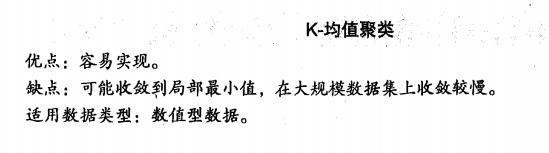K-均值是发现给定数据集的K个簇的算法。簇个数k是用户给定的，每一个簇通过质心，即簇中所有点的中心来描述。

首先，随机确定k个起始点为质心。然后将数据集中的每个点分配到一个簇中，具体来讲，为每个点找到距其最近的质心，并将其分配给该质心所对应的簇。这一步完成后，每个簇的质心更新为该簇所有点的平均值。

伪代码：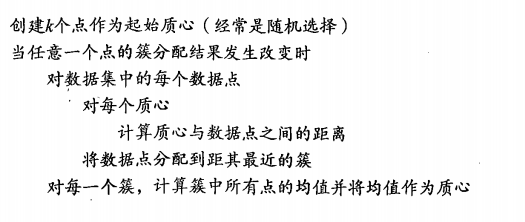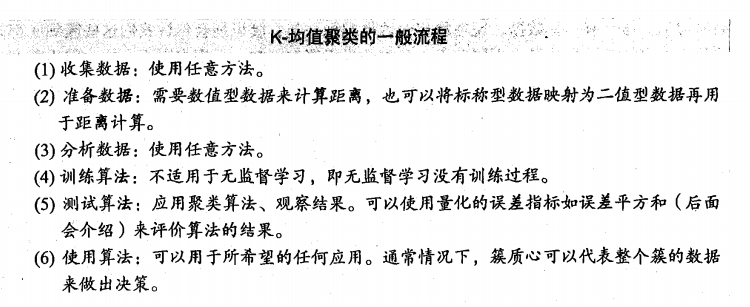下面给出K-均值算法的代码实现：

```from numpy import *

dataMat = []
fr = open(filename)
curLine = line.strip().split('\t')
fltLine = list(map(float, curLine))
#print(fltLine)
dataMat.append(fltLine)
return dataMat #返回一个包含许多列表的列表，容易将很多值封装到矩阵中

# 使用欧式距离计算两个向量的距离
def distEclud(vecA, vecB):
# 数组元素求平方
return sqrt(sum(power(vecA - vecB, 2))) #la.norm(vecA-vecB)

# 构建一个包含k个随机质心的集合
def randCent(dataSet, k):
n = shape(dataSet)
centroids = mat(zeros((k, n)))
for j in range(n):
'''
随机质心必须要整个数据集的边界之内
找到每个维的最大值和最小值，求出范围
然后生成0到1.0之间的随机数并通过最小值和取值范围，以便确保随机点在数据边界之内
'''
minJ = min(dataSet[:,j])
rangeJ = float(max(dataSet[:,j]) - minJ)
centroids[:,j] = mat(minJ + rangeJ * random.rand(k,1)) # 随机样本位于0到1中
return centroids
```

首先观察矩阵中的最大值与最小值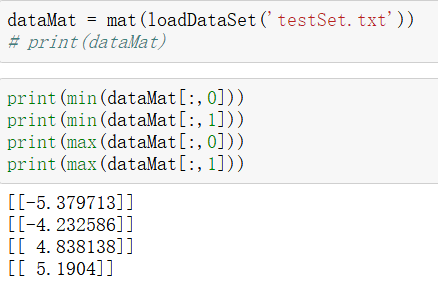然后看看randCent()函数能否生成min到max之间的值：从下图观察是可以的。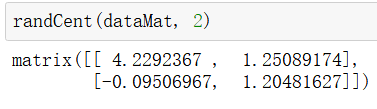再测试一下距离的计算方法：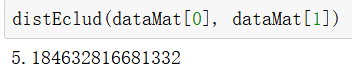所有的支持函数都可以正常运行。就可以开始实现k-均值算法了，该算法会创建k个质心，然后将每个点分配到距离最近的质心，再重新计算质心，重复数次，直到数据点的簇分配结果不再改变为止。

```def kMeans(dataSet, k, distMeas=distEclud, createCent=randCent):
'''
输入四个参数：数据集 k是必选参数，计算距离参数和创建初始质心参数是可选的
'''
m = shape(dataSet) # 数据集样本点数
# 簇分配结果矩阵 一列记录簇索引值 一列存储误差：指当前点到簇质心的距离
clusterAssment = mat(zeros((m,2)))
centroids = createCent(dataSet, k) # 创建k个质心
clusterChanged = True # 标志变量，值为true就继续迭代
while clusterChanged:
clusterChanged = False
for i in range(m):#for each data point assign it to the closest centroid
minDist = inf; minIndex = -1
for j in range(k):
distJI = distMeas(centroids[j,:],dataSet[i,:])
if distJI < minDist:
minDist = distJI; minIndex = j
if clusterAssment[i,0] != minIndex: clusterChanged = True
clusterAssment[i,:] = minIndex,minDist**2
print (centroids)
for cent in range(k):#recalculate centroids
ptsInClust = dataSet[nonzero(clusterAssment[:,0].A==cent)]#get all the point in this cluster
centroids[cent,:] = mean(ptsInClust, axis=0) # 沿矩阵列方向计算均值
return centroids, clusterAssment```

导入数据集运行结果如下：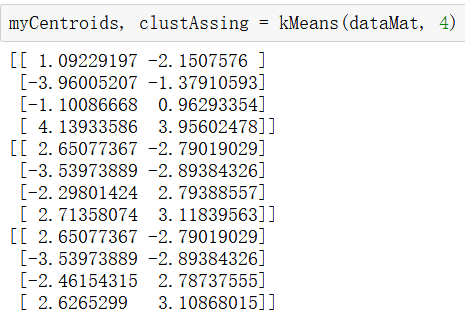上面结果给出了四个质心，可以看到经过3次迭代之后算法收敛。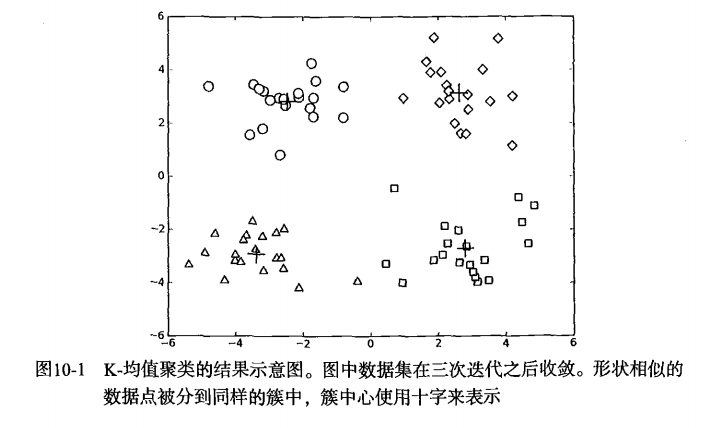## 2. 使用后处理来提高聚类性能

k是一个用户预先定义的参数，但是如何才能知道k的选择是否正确呢？如何才能知道簇的生成式比较好的呢？在包含簇分配结果的矩阵中保存着每个点的误差，即该点到簇质心的距离的平方值。我们会利用该误差来评价聚类质量的方法。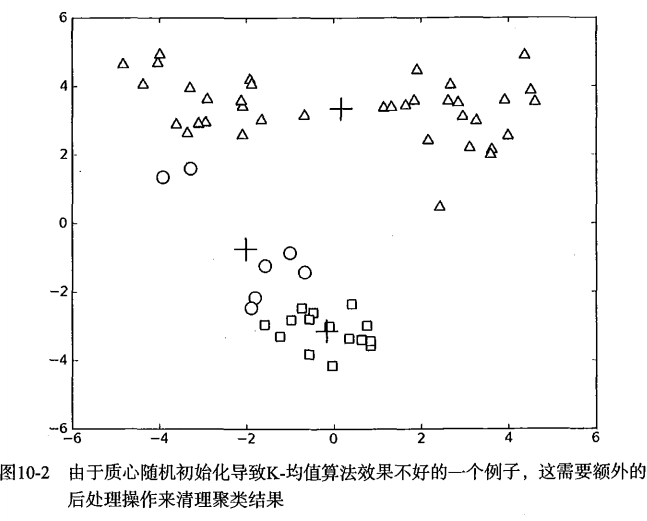图10-2的聚类结果，这是一个三个簇的数据集在运行了k均值算法之后的结果，但是点的簇分配结果并没有那么准确。k均值聚类算法比较差的原因是算法收敛到了局部最小值，而不是全局最小值。

一种度量聚类效果的指标是SSE（sum of squared error, 误差平方和），对应上面程序中clusterAssment矩阵的第一列之和。SSE值越小表示数据点越接近于它们的质心，聚类效果也就越好。因为对误差取了平方，所以更重视那些远离中心的点。

那么该怎么对10-2的结果进行改进？可以对生成的簇进行后处理，一种方法是将具有最大SSE值的簇划分成两个簇。具体实现时可以将最大簇包含的点过滤出来并在这些点上面进行k-均值算法，k=2。

为了保持簇总数不变，可以将某两个簇合并。从图中可以很明显的看出，要对下面两个出错的簇进行合并，但是我们这是在二维可视化的结果进行分析的，如果是四十维的数据应该如何处理？

有两种解决方法：合并最近的质心，或者合并两个使得SSE增幅最小的质心。第一种思路计算所有质心之间的距离，然后合并最近的两个点来实现；第二种方法需要合并两个簇然后计算总的SSE值。必须在所有可能的两个簇上重复上述的过程，直到找到合并最佳的两个簇为止。

## 3. 二分K-均值算法

为了克服k-均值算法收敛到局部最小值的问题，有人提出了另一个称为二分k-均值的算法。该算法首先将所有点作为一个簇，然后将簇一分为二。之后选择其中一个簇继续划分，选择哪一个簇进行划分取决于是否可以最大程度降低SSE的值。上述的划分福偶成不断重复，直到得到用户指定的簇数目为止。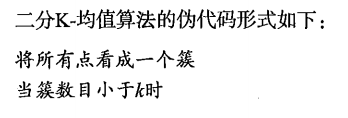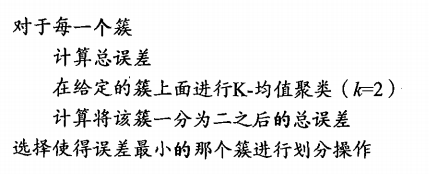另一种做法是选择SSE最大的簇进行划分，直到簇数目达到用户的指定数目，下面来实现以下实际效果：

```def biKmeans(dataSet, k, distMeas=distEclud):
m = shape(dataSet)
clusterAssment = mat(zeros((m,2))) # 存放分配结果及平方误差
centroid0 = mean(dataSet, axis=0).tolist()
centList =[centroid0] #列表 存放整个数据集的质心
for j in range(m):# 遍历数据集中所有的点来计算每个点到质心的误差值
clusterAssment[j,1] = distMeas(mat(centroid0), dataSet[j,:])**2
while (len(centList) < k):
lowestSSE = inf
for i in range(len(centList)):
ptsInCurrCluster = dataSet[nonzero(clusterAssment[:,0].A==i),:]#get the data points currently in cluster i
centroidMat, splitClustAss = kMeans(ptsInCurrCluster, 2, distMeas)
sseSplit = sum(splitClustAss[:,1])#compare the SSE to the currrent minimum
sseNotSplit = sum(clusterAssment[nonzero(clusterAssment[:,0].A!=i),1])
print ("sseSplit, and notSplit: ",sseSplit,sseNotSplit)
if (sseSplit + sseNotSplit) < lowestSSE:
bestCentToSplit = i
bestNewCents = centroidMat
bestClustAss = splitClustAss.copy()
lowestSSE = sseSplit + sseNotSplit
bestClustAss[nonzero(bestClustAss[:,0].A == 1),0] = len(centList) #change 1 to 3,4, or whatever
bestClustAss[nonzero(bestClustAss[:,0].A == 0),0] = bestCentToSplit
print ('the bestCentToSplit is: ',bestCentToSplit)
print ('the len of bestClustAss is: ', len(bestClustAss))
centList[bestCentToSplit] = bestNewCents[0,:].tolist()#replace a centroid with two best centroids
centList.append(bestNewCents[1,:].tolist())
clusterAssment[nonzero(clusterAssment[:,0].A == bestCentToSplit),:]= bestClustAss#reassign new clusters, and SSE
return mat(centList), clusterAssment```

运行结果：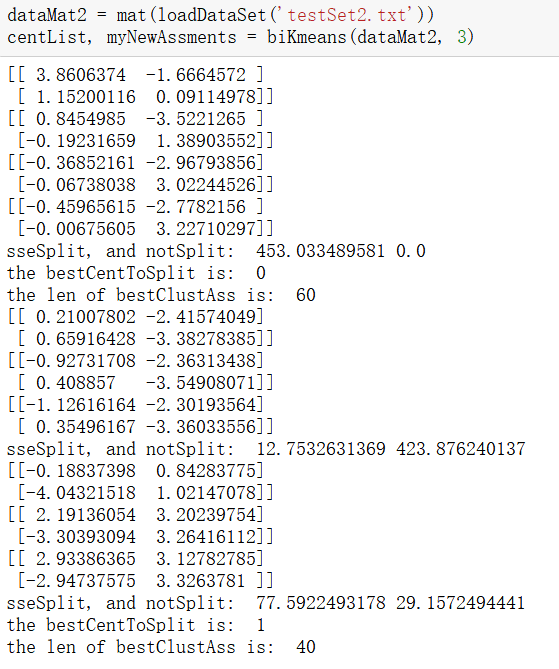质心：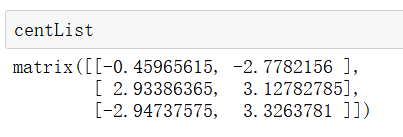上述函数可以运行多次，聚类会收敛到全局最小值，而原始的kMeans()会陷入局部最小值。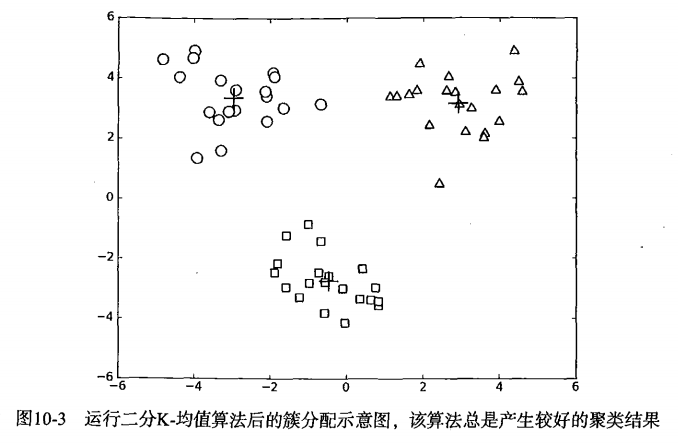## 4.本章小结

k-均值聚类是一种广泛的使用方法，以k个随机质心开始，算法会计算每个点到质心的距离，每个点会被分配到距其最近的簇质心，然后基于新分配到簇的点更新簇质心。以上过程重复数次，直到簇质心不再改变。这个方法非常简单有效，但是会受到初始簇质心的影响，为了获得更好的聚类效果可以使用另一种二分k-均值聚类算法。二分k-均值算法首先将所有点作为一个簇，然后使用k-均值聚类算法（k=2）对其划分。下一次迭代时，选择有最大误差的簇进行划分。该过程重复，直到k个簇创建成功为止。

k均值 机器学习实战 相关内容